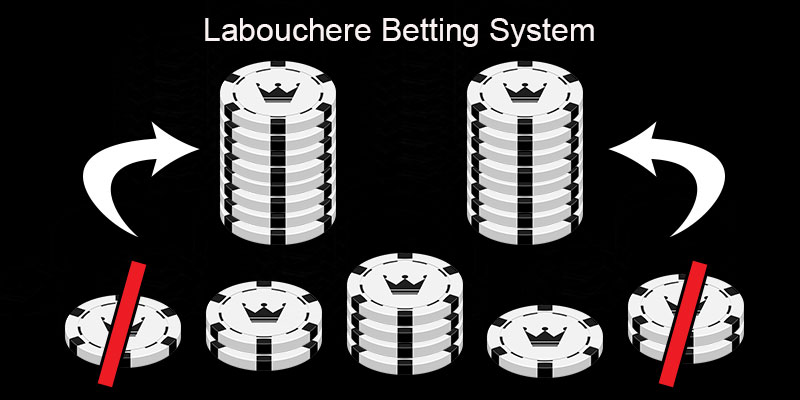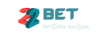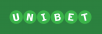July 2, 2022## Top 10 online bookmakers with the best odds

 Bookmaker Website Rating Welcome Bonus Play1xbet.com 9.4/10https://in.1xbet.com ₹ 7500 PLAYbetrally.com 8.0/10 ₹ 7500 PLAYspinpalacesports.com 7.8/10 €200 PLAYbet365.com 9.5/10 ———— PLAY888sport.com 8.6/10 €100 PLAYbetfair.com 9.2/10 \$30 PLAY22bet.com 7.8/10 €100 PLAYunibet.com 8.7/10 €25 PLAYdafabet.com 8.0/10 INR 10.000 PLAY18bet.com 7.8/10 125 PLAYfavbet.com 7.6/10 €10 PLAY

# How to use the Labouchere System on online sports bettingWhat’s the ideal winning percentage you have to hit in order to gain an acceptable profit? There are a lot of answers to this question, some of them come directly from mathematical sequences or even from roulette strategies.

The Labouchere strategy is one of the most popular methods of determining the stake of your next bet and is often referred as one of the best roulette strategies. You might have bumped into it under one of the following names: Cancellation System, Split Martingale, American Progression, American Roulette Cancellation and Montante Americaine. Despite their different names, they all describe the same, successful betting system.

Rumour has it that this strategy was invented by Henry Labouchere (1831-1912), an English aristocrat and keen roulette player. However there has been evidence of it been used 100 years before his time, in various casino games of 18th century France. However, it was under his name that the strategy became one of the best known roulette and eventually betting systems, along with Martingale.

### Where to use the Labouchere football system?

The Labouchere system is better used in 2-way markets on evenly offered odds (1/1 or 2.00) or real close to even (over 1.90). In football betting the ideal markets are Asian Handicaps or other special 2-way market bets (over/under, goal/no goal etc.). Even in roulette, the theoretical 50%-50% chances are not totally accurate, due to the presence of the “0” number.

The main idea behind the Labouchere progression system is to try and surpass a sequence of losses with the minimum number of wins possible. This idea is almost the same with the Martingale system. After all, both systems are based on “negative sequences” and demand an increase of the stake after a losing bet.

The main difference between them is that while the Martingale tries to recoup previous losses on your followingbet, the Labouchere has a far more bland approach: trying to gain profit from a sequence of prospected winning bets.

### The winning recipe

You can use the Labouchere football system by following these simple steps:

How much money do you want to win when the sequence ends? All you have to do is choose a number and start making sequenced additions, by adding your initial number to the next number you find. The sum of all your additions will be your estimated ideal profit.

Need an example? Here are two.

Chosen number: 1

Sequence: 1+2+3+4+5+6+7 (ideal profit= €28)

Chosen number: 2

Sequence: 2+4+6+8+10+12+14+16+18+20 (ideal profit= €110)

You can choose any number you want and continue adding numbers whenever you like. However, keep in mind that the greater the numbers or the sequences are, the more money you have to risk every time, to gain your ideal profit. This means it might get harder for you to follow the sequence. You’re strongly recommended to start with low sums, especially on your first tries.

Let’s focus on the first example: When you start using the Labouchere system, you simply add the FIRST and LASTnumber of the sequence and stake the sum (1+7= €8). The first and last number depend on the sequence of wins and losses and change every time as follows:

• If the first bet is won, you ABSTRACT the first and the last number of your initial sequence and sum the NEW first and last number.

• If the first bet is lost, you ADD the sum you staked at the end of the sequence and sum the NEW first and last number for your next bet.

A sequence example

With this example we can watch the Labouchere football betting system in action. Suppose we stake all bets on 2.00 odds.

 # Sequence Stake Result New Sequence Bankroll 1 1+2+3+4+5+6+7 8 Loss 1+2+3+4+5+6+7+8 -8 2 1+2+3+4+5+6+7+8 9 Loss 1+2+3+4+5+6+7+8+9 -17 3 1+2+3+4+5+6+7+8+9 10 Win 2+3+4+5+6+7+8 -7 4 2+3+4+5+6+7+8 10 Loss 2+3+4+5+6+7+8+10 -17 5 2+3+4+5+6+7+8+10 12 Loss 2+3+4+5+6+7+8+10+12 -29 6 2+3+4+5+6+7+8+10+12 14 Win 3+4+5+6+7+8+10 -15 7 3+4+5+6+7+8+10 13 Loss 3+4+5+6+7+8+10+13 -28 8 3+4+5+6+7+8+10+13 16 Loss 3+4+5+6+7+8+10+13+16 -44 9 3+4+5+6+7+8+10+13+16 19 Win 4+5+6+7+8+10+13 -25 10 4+5+6+7+8+10+13 17 Win 5+6+7+8+10 -8 11 5+6+7+8+10 15 Win 6+7+8 +7 12 6+7+8 14 Loss 6+7+8+14 –7 13 6+7+8+14 20 Win 7+8 +13 14 7+8 15 Win End of sequence +28

In the above 14 bets, you risked €192 in total. A €28 profit means that you hit a 14,5% yield, which is one of the highest you can reach in the long term.What’s the point of this? With only a 50% winning percentage (7 winning out of 14 total bets), which is not that difficult to hit, and low risk, stake-wise, you can earn an acceptable profit.

### Play safe or aggressivelyAs you can see, Labouchere is a very flexible betting system, allowing you to determine your bankroll and risk at will. However, there are some variations that make it either safer or riskier, according to the punter’s playstyle.

Zero Labouchere: Some punters, especially when testing the Labouchere betting systemlike to start with a lot of zero numbers at the beginning of the sequence. When they “build” their sequence, it looks like this: 0+0+0+0+0+1+2+3+4+5 etc. The more zeros there are, the safer the system is, as after every loss, you have to stake the same amount on your next bet, as per the number of zeros.

Dividing Labouchere: When your winning percentage in a Labouchere system session is less than 40%, there’s always the danger of placing continuous high stakes betsIn order to avoid this scenario some punters divide the advised stake by 2, when it exceeds the ideal profit amount.

For example, let’s say you follow a Labouchere sequence 1+2+3+4+5+6+7 with an ideal profit of €28. You have found yourself at bet#24 and are advised to place €30 on your next bet. If you don’t feel like risking that much, you could divide your stake at half: 30/2= €15. The only drawback, is that you now have to reach an even better winning percentage to match your losses.

Half Labouchere: This is a rather aggressive strategy. The stake of your bet, should be the sum of the LASTnumbers of the sequence divided by two (Half). You can choose how many numbers to add, according to how much you want to risk.

An example with a four digit sequence (1+2+3+4), adding all (4) of them.

 # Sequence Stake Result New Sequence Bankroll 1 (1+2+3+4)/2 5 Loss 1+2+3+4+5 -5 2 (2+3+4+5)/2 7 Loss 1+2+3+4+5+8 -12 3 (3+4+5+8)/2 10 Loss 1+2+3+4+5+8+10 -22 4 (4+5+8+10)/2 14 Win 2+3+4+5+8 -8 5 (3+4+5+8)/2 10 Loss 2+3+4+5+8+10 -18 6 (4+5+8+10)/2 14 Win 3+4+5+8 -4 7 (3+4+5+8)/2 10 Win 4+5 +6 8 (4+5)/2 5 Win End of Sequence +11

#### Pros and cons of Reverse Labouchere

This is by far the most “aggressive” variation of the system, as the main idea is applied from the absolute opposite side. In this case you ADD the sum at the end of the sequence when you WIN and you ABSTRACT the first and the last number of the sequence when you LOSE. The risk is bigger, as the winning percentage required is far larger(more than 60%).However the profit is much higher as well.

• The main advantage of the Labouchere betting system is that you can earn a
•  profit with a winning percentage lower than 50%.
• There aren’t any confusing mathematical types, you simply add or abstract. Even a punter with minimum math skills can follow Labouchere.
• Even on weekdays, consecutive bets are available, due to the huge number of games offered by online bookmakers.
• As in any other negative sequence theory, the Labouchere system can be very helpful when the stakes are not extremely high, meaning that you can afford some consecutive losses.
• Your available bankroll must always greatly exceed your ideal profit, before you start a Labouchere sequence. In any other case, you’re in danger of high capital loss. Theoretically, you need a lot of money to follow, but you can start with small stakes.
• If you bet high (for example, following a 25+50+75+100+125+150 sequence) you might face problems with certain online bookies limiting your bets. Especially in high stakes you should double check the limits oneach match you’re about to bet.
• Discipline is worth its weight in gold in every betting system. If you try to change the system in the middle of a session, the most possible scenario is that you’ll lose all your money